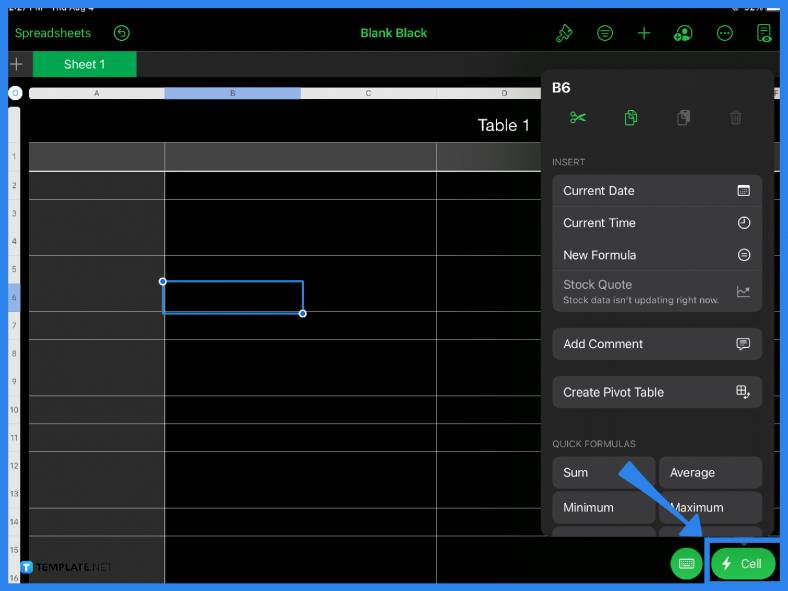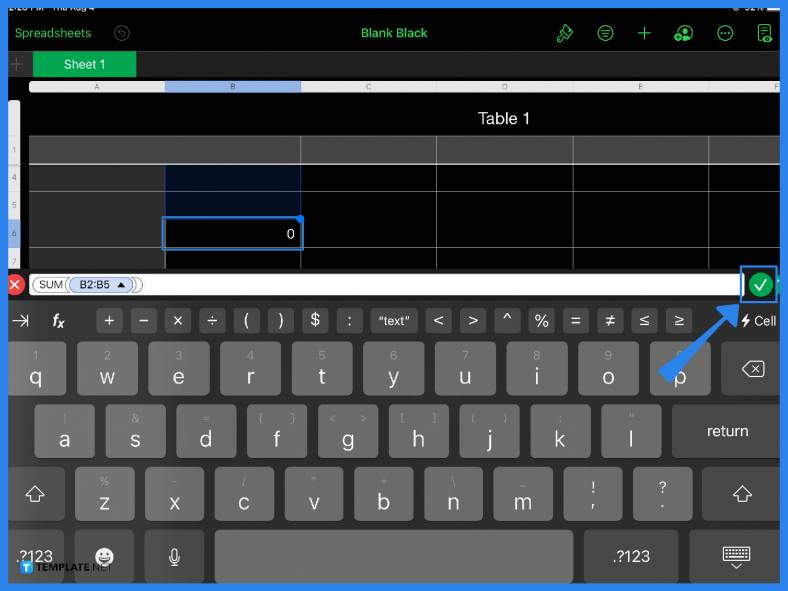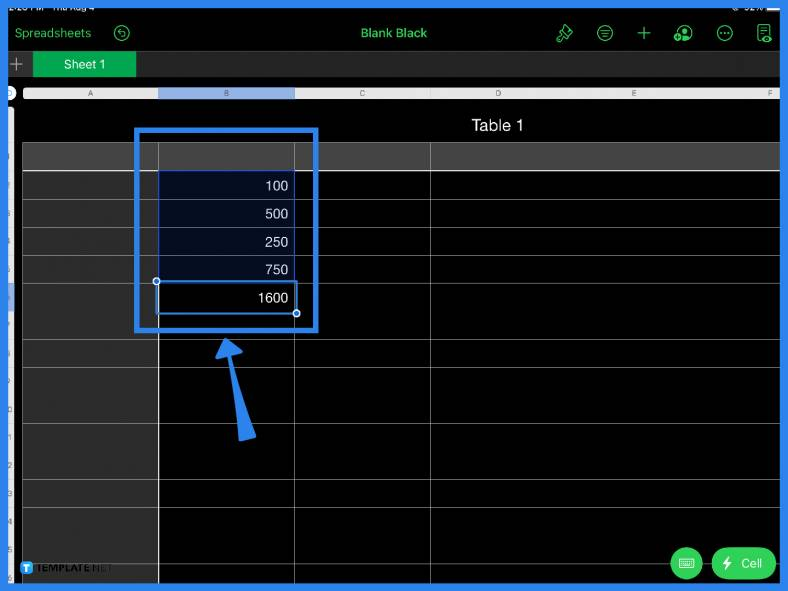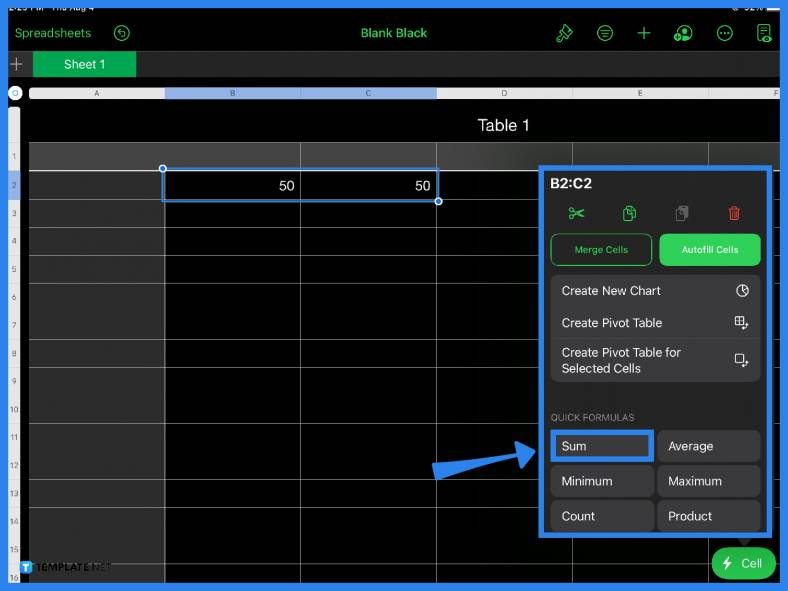# How to Use Sum Function in Apple Numbers

Addition is the most basic mathematical function. Apple Numbers offers an easy sum function and a host of other different functions to make it more convenient for users to calculate mathematical equations.## How to Use Sum Function in Apple Numbers

Apple Numbers has a sum function that you can use to add numbers and values instantly. Follow the step-by-step tutorial guide below to know how to properly use and execute this function.

• ### Step 1: Choose a Cell to FormatThe first step is to pick a cell to place or put your sum. Click on the Cell button located in the lower right corner of the page. Under Quick Formulas, select the Sum function. This sets the formula of the cell, even if you have not placed any numbers yet on the spreadsheet. You can choose any cell in your spreadsheet, depending on your objectives or the specific content of your sheet.

• ### Step 2: Use the Formula BarIf you click on any cell and type in the equal sign (=), you will notice that the formula bar immediately appears. You can also use the formula bar and its addition function (+) to get the sum. Just type in the numbers you want to add and the arithmetic operator. In this case, use the plus (+) sign. Click on the green check mark and you will immediately get the sum.

• ### Step 3: Add ValuesAlternatively, you can also simply add values or numbers to your spreadsheet while making sure the cells match the one in your formula. Doing this will still give you the same result. So whether you need to craft an income and expense sheet, audit report, revenue projection, inventory report, or even a simple budget checklist, Apple Numbers makes addition quick and easy.

• ### Step 4: Click and Drag NumbersIf you simply want to use the spreadsheet as a quick calculator, just type your numbers in and highlight the cells then tap the Cell icon and select the Sum function from the Quick Formulas menu.

## FAQs

### Where is the sum button in Apple Numbers?

The sum function button can be found under Quick Formulas when you open the Cell Action menu.

### How do you sum a column in Apple Numbers?

To add up all the values of an entire column, highlight the column and select the Sum function under Quick Formulas found in the Cell menu.

### How do you write a sum formula in Apple Numbers?

To write a sum formula, click on a cell, type in the equal (=) sign, input the first value, add the arithmetic operator or the addition (+) sign, add the other values, and tap Enter.

### How do you calculate in Apple Numbers on a Mac?

For Mac users, you can perform various mathematical calculations in Apple Numbers by using the equal (=) sign and choosing an arithmetic operator (e.g., +, -, /) from the formula bar.

### What does the Sum function do?

In Apple Numbers, the Sum function adds values or numbers from individual cells, entire rows, columns, cell references, or a combination of all of these.